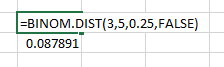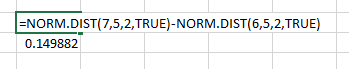# How to compute probabilities from a distribution

## Description

There are many famous continuous probability distributions, such as the normal and exponential distributions. How can we get access to them in software, to compute the probability of a value/values occurring?

## Solution, in Excel

View this solution alone.

If the probability distribution is a common discrete distribution, you can simply use a built-in function from Excel’s set of statistical functions to compute any probability from it.

For example, to find the probability that a binomial random variable with p=0.25 yields 3 successes in 5 trials, you can use =BINOM.DIST(3,5,0.25,FALSE). The final parameter, FALSE, tells Excel you are asking only about 3 successes, not the cumulative probability of up to 3 successes.For other discrete random variables, see the Excel help on POISSON.DIST and HYPGEOM.DIST.

If the probability distribution is a common continuous distribution, you must ask about the probability of a random value falling in a certain range. You do so by subtracting two outputs of the cumulative distribution function (CDF).

For example, to find the probability that a normal random variable with mean 5 and standard deviation 2 falls in the interval [6,7], you can use =NORM.DIST(7,5,2,TRUE)-NORM.DIST(6,5,2,TRUE). Notice:

• It is important to subtract the lower end of the interval from the higher end, not the other way around. (If your probability comes out negative, you have it backwards.)

• The final parameter, TRUE, tells Excel you are using the CDF of the distribution. If you use FALSE instead, you will get a wrong answer.For other continuous random variables, see the Excel help on BETA.DIST, CHISQ.DIST, F.DIST, GAMMA.DIST, LOGNORM.DIST, and T.DIST.

See a problem? Tell us or edit the source.

## Solution, in Julia

View this solution alone.

You can import many different random variables from Julia’s Distributions package. The full list of them is online here.

If you don’t have that package installed, first run using Pkg and then Pkg.add( "Distributions" ) from within Julia.

To compute a probability from a discrete distribution, create a random variable, then use the pdf function. (This is a slight misnomer, because PDF stands for Probability Density Function, which is a concept related to continuous random variables, but it’s the function Julia uses.)

1
2
3
4
5
6
7
8
using Distributions

# Create a binomial random variable with 10 trials
# and probability 0.5 of success on each trial
X = Binomial( 10, 0.5 )

# What is the probability of exactly 3 successes?
pdf( X, 3 )

1
0.1171875000000004


To compute a probability from a continuous distribution, create a random variable, then use its Cumulative Density Function, cdf. You can only compute the probability that a random value will fall in an interval $[a,b]$, not the probability that it will equal a specific value.

1
2
3
4
5
6
7
using Distributions

# Create a normal random variable with mean μ=10 and standard deviation σ=5
X = Normal( 10, 5 )

# What is the probability of the value lying in the interval [12,13]?
cdf( X, 13 ) - cdf( X, 12 )

1
0.07032514063960227


See a problem? Tell us or edit the source.

## Using SciPy, in Python

View this solution alone.

You can import many different random variables from SciPy’s stats module. The full list of them is online here.

To compute a probability from a discrete distribution, create a random variable, then use its Probability Mass Function, pmf.

1
2
3
4
5
6
7
8
from scipy import stats

# Create a binomial random variable with 10 trials
# and probability 0.5 of success on each trial
X = stats.binom( 10, 0.5 )

# What is the probability of exactly 3 successes?
X.pmf( 3 )

1
0.1171875


To compute a probability from a continuous distribution, create a random variable, then use its Cumulative Density Function, cdf. You can only compute the probability that a random value will fall in an interval $[a,b]$, not the probability that it will equal a specific value.

1
2
3
4
5
6
7
from scipy import stats

# Create a normal random variable with mean μ=10 and standard deviation σ=5
X = stats.norm( 10, 5 )

# What is the probability of the value lying in the interval [12,13]?
X.cdf( 13 ) - X.cdf( 12 )

1
0.07032514063960227


See a problem? Tell us or edit the source.

## Solution, in R

View this solution alone.

Because R is designed for use in statistics, it comes with many probability distributions built in. A list of them is online here.

To compute a probability from a discrete distribution, prefix the name of the distribution with d (for “density”) and call it as a function on the value whose probability you want to know, plus any parameters the distrubtion needs.

1
2
3
4
# For a binomial random variable with 10 trials
# and probability 0.5 of success on each trial,
# what is the probability of exactly 3 successes?
dbinom( 3, size=10, prob=0.5 )

1
 0.1171875


If you change the prefix to p, then R will compute the probability up to the parameter you specify, as in the following example.

1
2
3
4
# For a binomial random variable with 10 trials
# and probability 0.5 of success on each trial,
# what is the probability of up to (and including) 3 successes?
pbinom( 3, size=10, prob=0.5 )

1
 0.171875


To compute a probability from a continuous distribution, prefix the name with d, just as in the example above. But you can compute only the probability that a random value will fall in an interval $[a,b]$, not the probability that it will equal a specific value.

1
2
3
# For a normal random variable with mean μ=10 and standard deviation σ=5,
# what is the probability of the value lying in the interval [12,13]?
pnorm( 13, mean=10, sd=5 ) - pnorm( 12, mean=10, sd=5 )

1
 0.07032514


Consequently, we can also compute:

1
2
pnorm( 13, mean=10, sd=5 )     # the probability of a value < 13
1 - pnorm( 13, mean=10, sd=5 ) # the probability of a value > 13

1
2
3
4
5
 0.7257469

 0.2742531